Скачать презентацию Nature of Science The International System of Units

2c225819d091e2a7bbb4e68bfcdec57f.ppt

• Количество слайдов: 24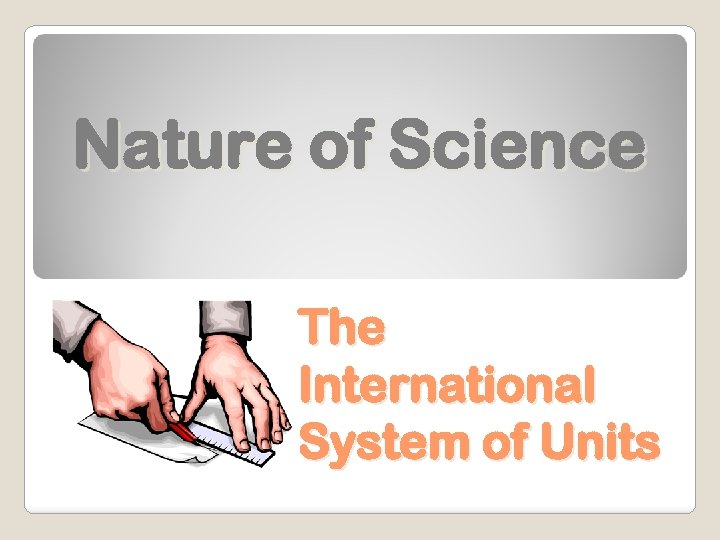Nature of Science The International System of Units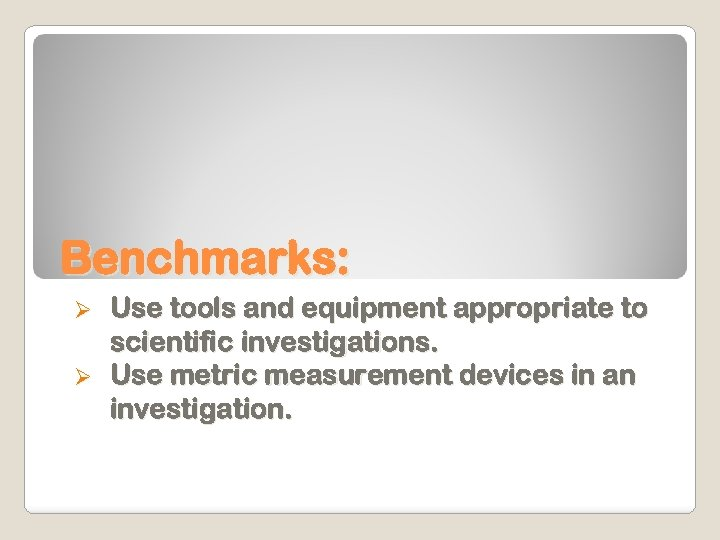Benchmarks: Use tools and equipment appropriate to scientific investigations. Ø Use metric measurement devices in an investigation. Ø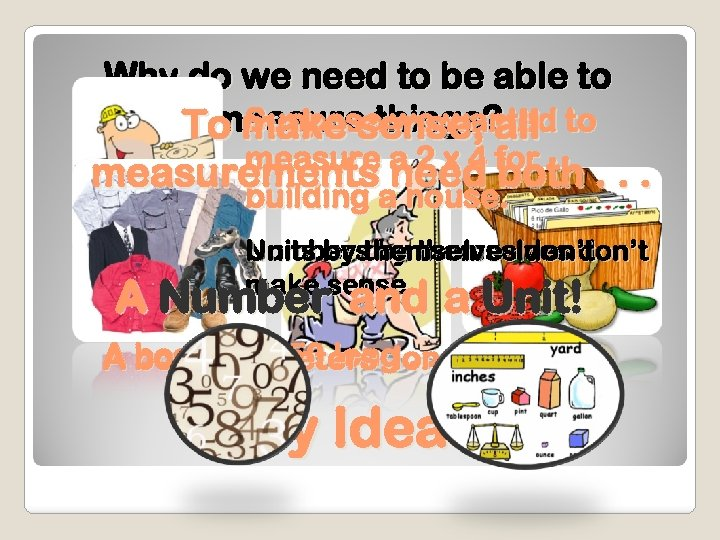Why do we need to be able to Suppose we wanted measure things? To make sense, all to measure a 2 x 4 for measurements need both. . . building a house. Units by themselves don’t Numbers by themselves don’t make sense. A Number and a Unit! A board is 350 long. . . . meters long Any Ideas?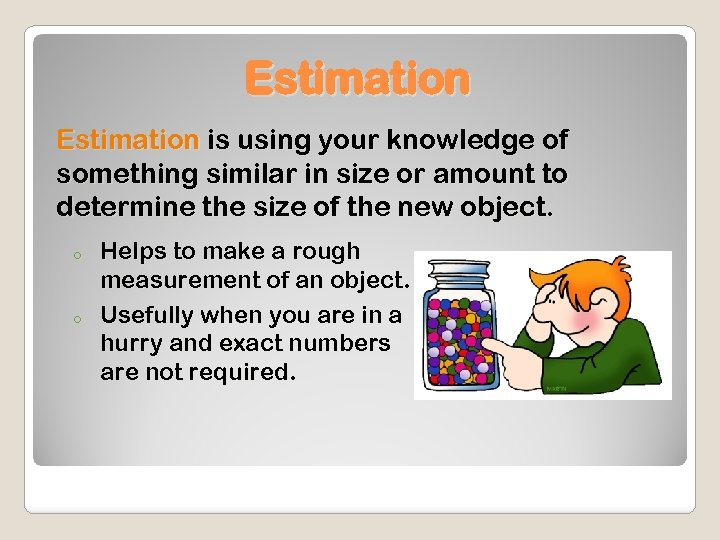Estimation is using your knowledge of something similar in size or amount to determine the size of the new object. o o Helps to make a rough measurement of an object. Usefully when you are in a hurry and exact numbers are not required.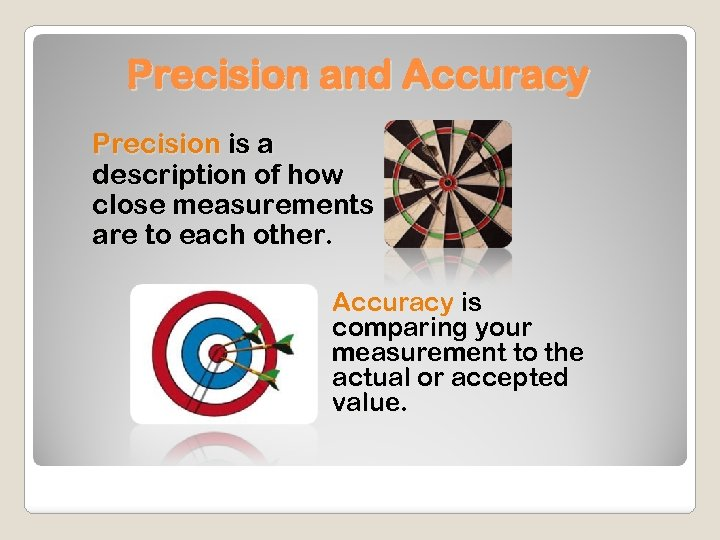Precision and Accuracy Precision is a description of how close measurements are to each other. Accuracy is comparing your measurement to the actual or accepted value.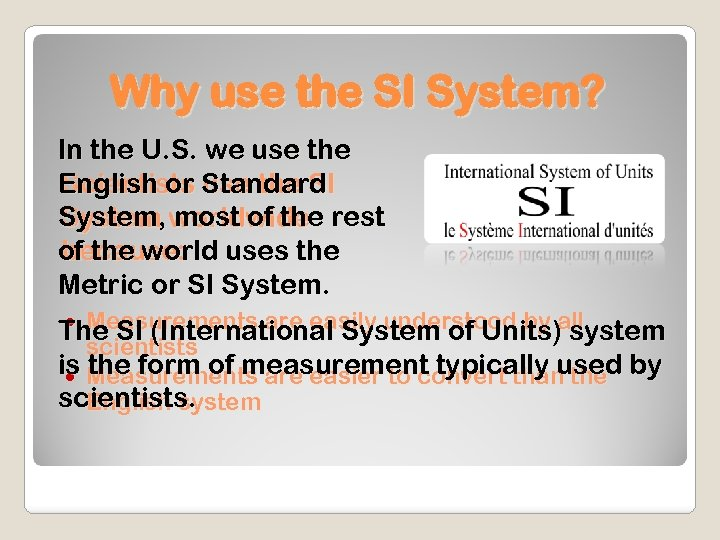Why use the SI System? In the U. S. we use the English or Standard Scientists use the SI System, worldwide rest System most of the world uses the because: Metric or SI System. Measurements are easily understood by The SI (International System of Units)all system scientists is Measurements are easier to convert than the by the form of measurement typically used scientists. English system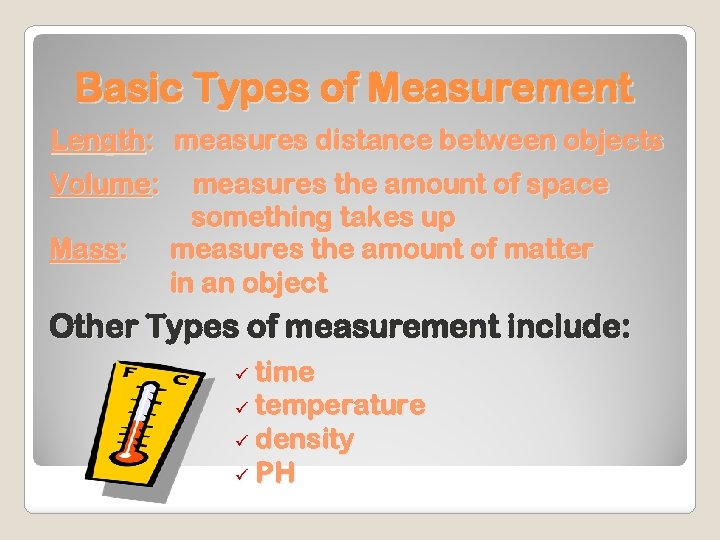Basic Types of Measurement Length: measures distance between objects Volume: Mass: measures the amount of space something takes up measures the amount of matter in an object Other Types of measurement include: time ü temperature ü density ü PH ü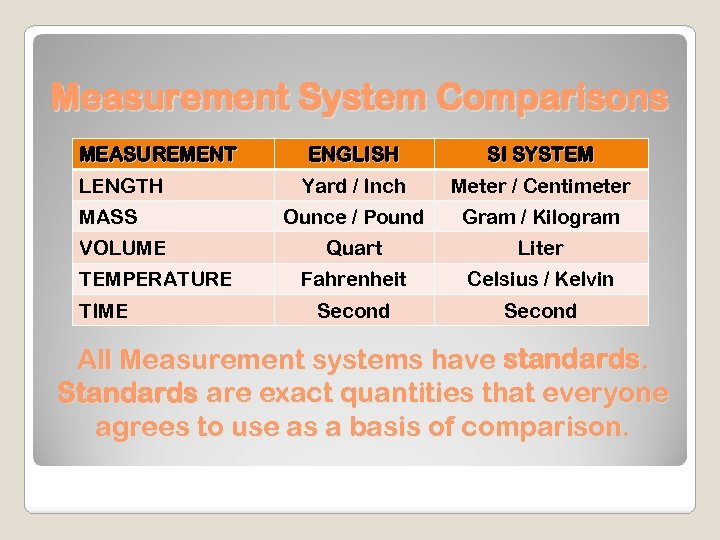Measurement System Comparisons MEASUREMENT LENGTH MASS VOLUME TEMPERATURE TIME ENGLISH SI SYSTEM Yard / Inch Meter / Centimeter Ounce / Pound Gram / Kilogram Quart Liter Fahrenheit Celsius / Kelvin Second All Measurement systems have standards. Standards are exact quantities that everyone agrees to use as a basis of comparison.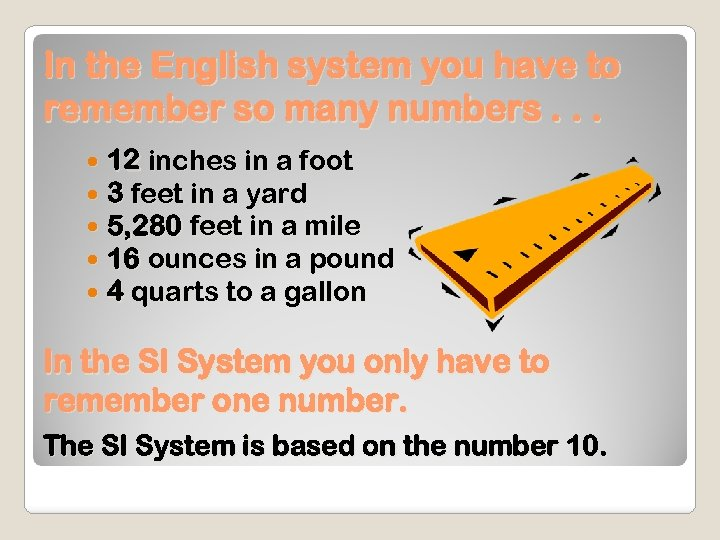In the English system you have to remember so many numbers. . . 12 inches in a foot 3 feet in a yard 5, 280 feet in a mile 16 ounces in a pound 4 quarts to a gallon In the SI System you only have to remember one number. The SI System is based on the number 10.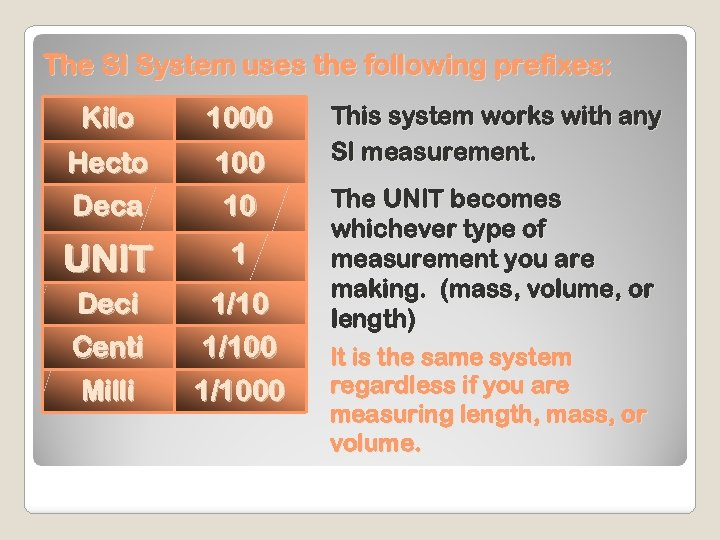The SI System uses the following prefixes: Kilo 1000 Hecto Deca 100 10 UNIT 1 Deci 1/10 Centi Milli 1/1000 This system works with any SI measurement. The UNIT becomes whichever type of measurement you are making. (mass, volume, or length) It is the same system regardless if you are measuring length, mass, or volume.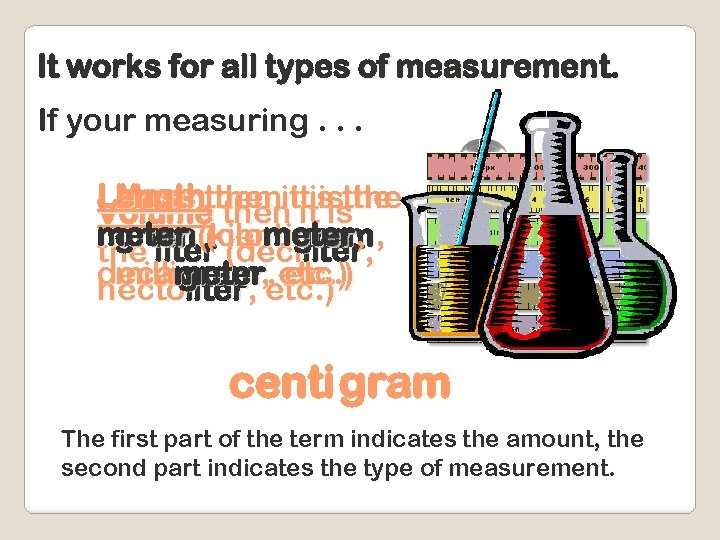It works for all types of measurement. If your measuring. . . Lengththen ititisthe Mass then itis the Volume then is meter (kilometer, , , gram (centigram the liter (deciliter decameter, etc. ) milligram , etc. ) hectoliter, centigram The first part of the term indicates the amount, the second part indicates the type of measurement.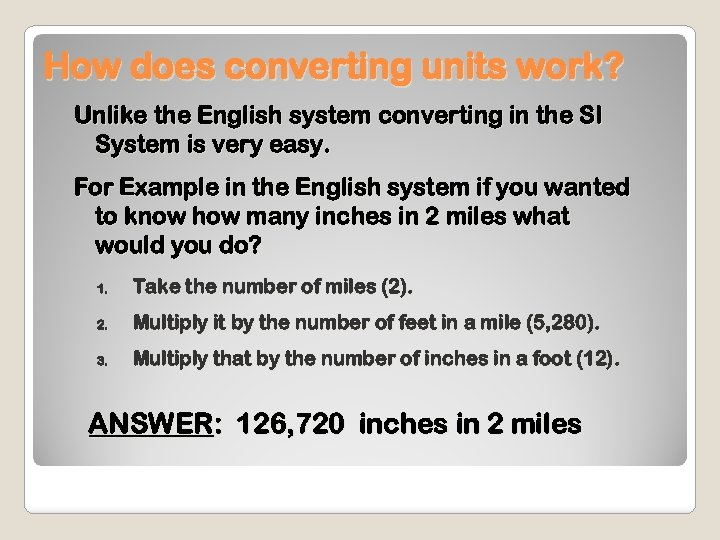How does converting units work? Unlike the English system converting in the SI System is very easy. For Example in the English system if you wanted to know how many inches in 2 miles what would you do? 1. Take the number of miles (2). 2. Multiply it by the number of feet in a mile (5, 280). 3. Multiply that by the number of inches in a foot (12). ANSWER: 126, 720 inches in 2 miles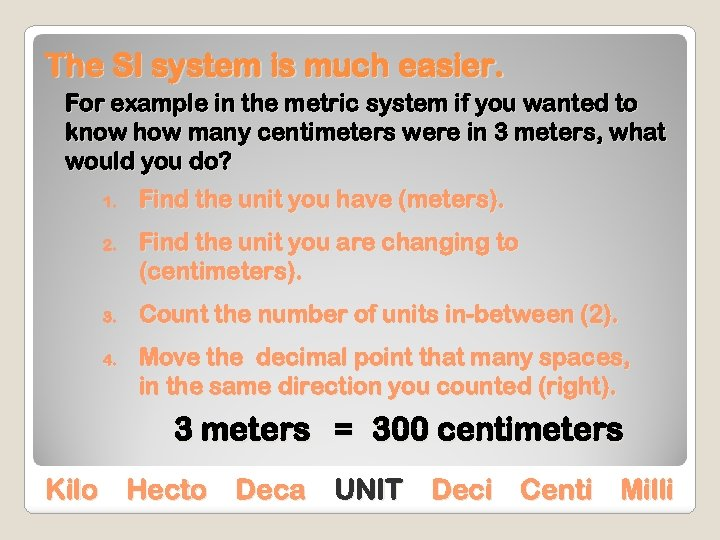The SI system is much easier. For example in the metric system if you wanted to know how many centimeters were in 3 meters, what would you do? 1. 2. 3. 4. Find the unit you have (meters). Find the unit you are changing to (centimeters). Count the number of units in-between (2). Move the decimal point that many spaces, in the same direction you counted (right). 3 meters = 300 centimeters Kilo Hecto Deca UNIT Deci Centi Milli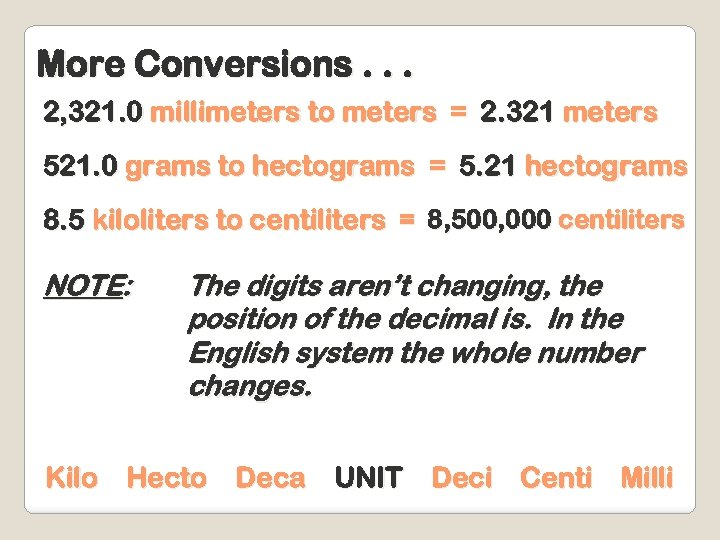More Conversions. . . 2, 321. 0 millimeters to meters = 2. 321 meters 521. 0 grams to hectograms = 5. 21 hectograms 8. 5 kiloliters to centiliters = 8, 500, 000 centiliters NOTE: The digits aren’t changing, the position of the decimal is. In the English system the whole number changes. Kilo Hecto Deca UNIT Deci Centi Milli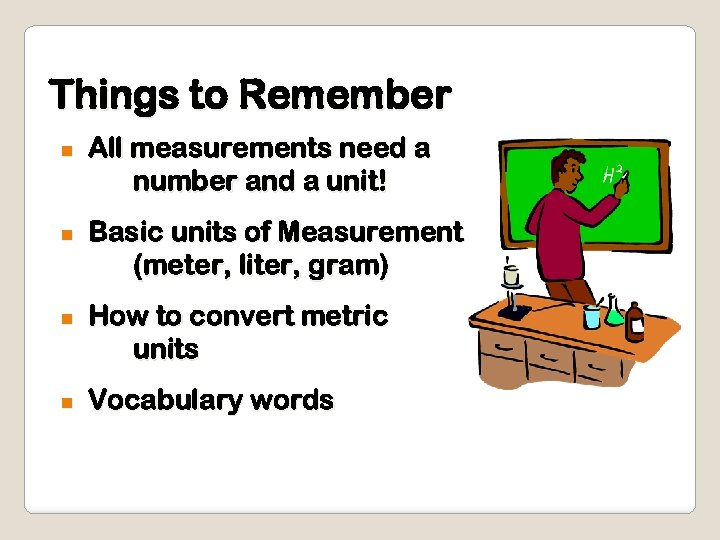Things to Remember n n All measurements need a number and a unit! Basic units of Measurement (meter, liter, gram) How to convert metric units Vocabulary wordsNature of Science The International System of Units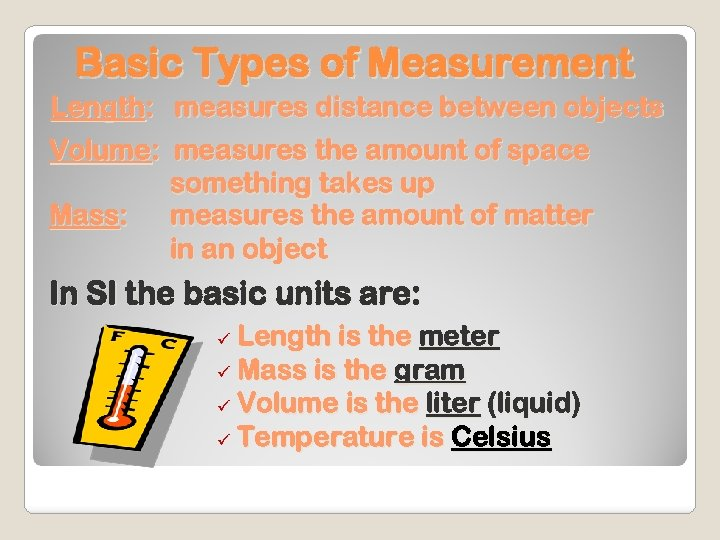Basic Types of Measurement Length: measures distance between objects Volume: measures the amount of space something takes up Mass: measures the amount of matter in an object In SI the basic units are: Length is the meter ü Mass is the gram ü Volume is the liter (liquid) ü Temperature is Celsius ü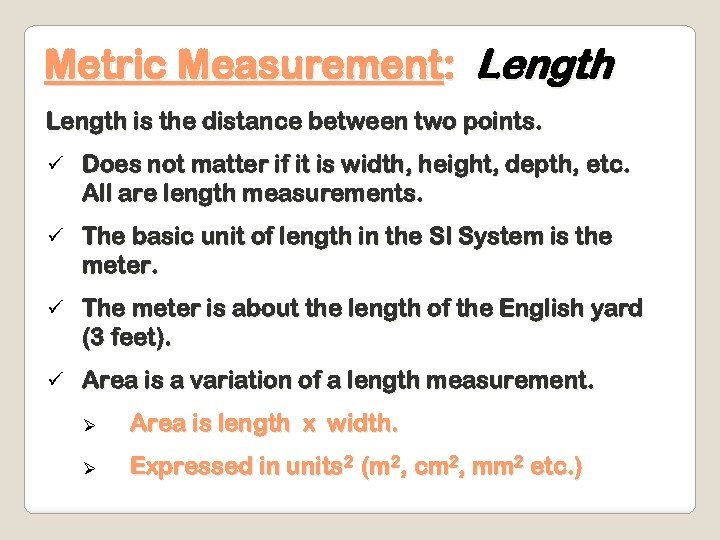Metric Measurement: Length is the distance between two points. ü Does not matter if it is width, height, depth, etc. All are length measurements. ü The basic unit of length in the SI System is the meter. ü The meter is about the length of the English yard (3 feet). ü Area is a variation of a length measurement. Ø Area is length x width. Ø Expressed in units 2 (m 2, cm 2, mm 2 etc. )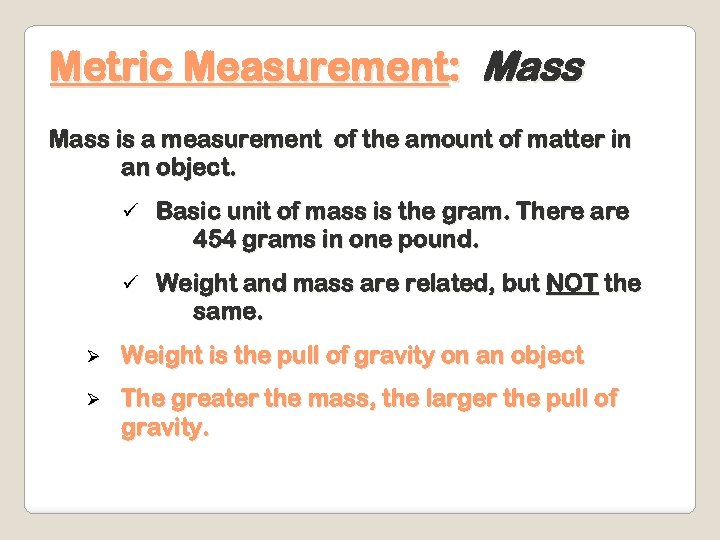Metric Measurement: Mass is a measurement of the amount of matter in an object. ü Basic unit of mass is the gram. There are 454 grams in one pound. ü Weight and mass are related, but NOT the same. Ø Weight is the pull of gravity on an object Ø The greater the mass, the larger the pull of gravity.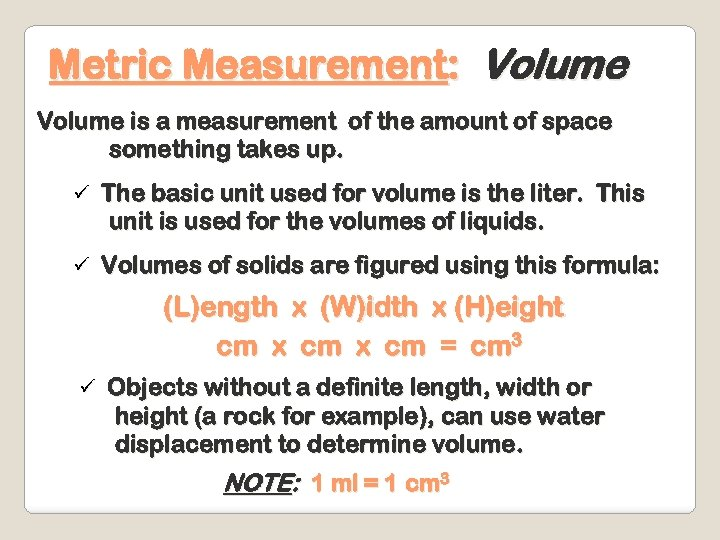Metric Measurement: Volume is a measurement of the amount of space something takes up. ü The basic unit used for volume is the liter. This unit is used for the volumes of liquids. ü Volumes of solids are figured using this formula: (L)ength x (W)idth x (H)eight cm x cm = cm 3 ü Objects without a definite length, width or height (a rock for example), can use water displacement to determine volume. NOTE: 1 ml = 1 cm 3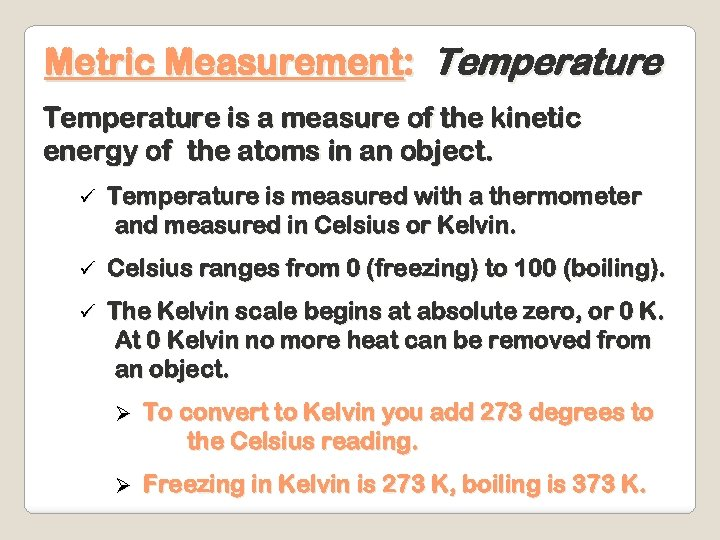Metric Measurement: Temperature is a measure of the kinetic energy of the atoms in an object. ü Temperature is measured with a thermometer and measured in Celsius or Kelvin. ü Celsius ranges from 0 (freezing) to 100 (boiling). ü The Kelvin scale begins at absolute zero, or 0 K. At 0 Kelvin no more heat can be removed from an object. Ø To convert to Kelvin you add 273 degrees to the Celsius reading. Ø Freezing in Kelvin is 273 K, boiling is 373 K.Nature of Science The International System of Units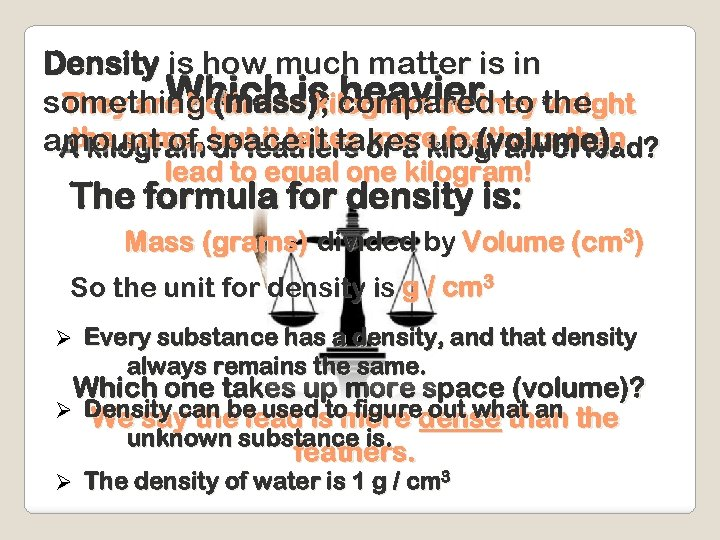Density is how much matter is in Which is heavier. weight something (mass)kilogram so they. They are both one , compared. to the same, of feathers more feathers than amount of space it takes kilogram of lead? A kilogram but it takes or a up (volume). lead to equal one kilogram! The formula for density is: Mass (grams) divided by Volume (cm 3) So the unit for density is g / cm 3 Ø Every substance has a density, and that density always remains the same. Which one takes up more space (volume)? Ø Density can be used to figuredense than the We say the lead is more out what an unknown substance is. feathers. Ø The density of water is 1 g / cm 3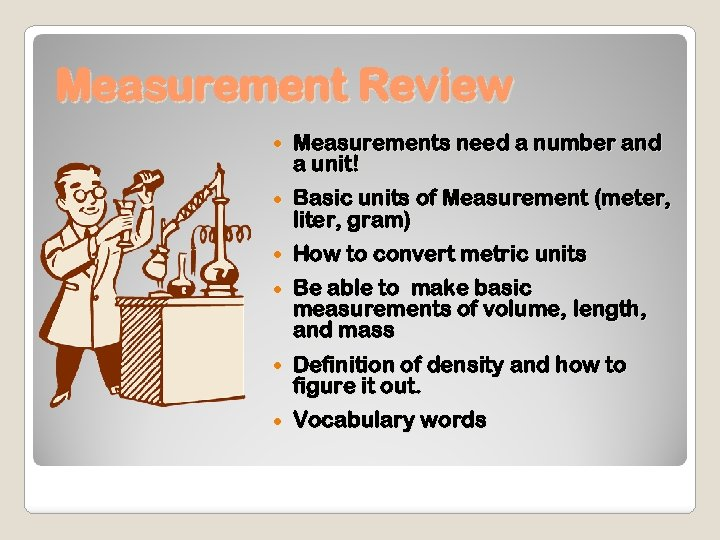Measurement Review Measurements need a number and a unit! Basic units of Measurement (meter, liter, gram) How to convert metric units Be able to make basic measurements of volume, length, and mass Definition of density and how to figure it out. Vocabulary words